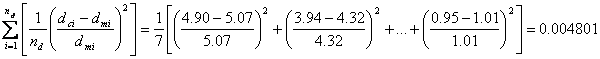# Backcalculation

“Backcalculation” is a mechanistic evaluation of pavement surface deflection basins generated by various pavement deflection devices. Backcalculation takes a measured surface deflection and attempts to match it (to within some tolerable error) with a calculated surface deflection generated from an identical pavement structure using assumed layer stiffnesses (moduli). The assumed layer moduli in the calculated model are adjusted until they produce a surface deflection that closely matches the measured one. The combination of assumed layer stiffnesses that results in this match is then assumed to be near the actual in situ moduli for the various pavement layers. The backcalculation process is usually iterative and normally done with computer software.

## Typical Flowchart

A basic flowchart (patterned after Lytton, 1989) that represents the fundamental elements in all known backcalculation programs is shown as Figure 1. Briefly, these elements include:

• Measured deflections. Includes the measured pavement surface deflections and associated distances from the load.
• Layer thicknesses and loads. Includes all layer thicknesses and load levels for a specific test location.
• Seed moduli. The seed moduli are the initial moduli used in the computer program to calculate surface deflections. These moduli are usually estimated from user experience or various equations.
• Deflection calculation. Layered elastic computer programs are generally used to calculate a deflection basin.
• Error check. This element simply compares the measured and calculated basins. There are various error measures which can be used to make such comparisons (more on this in a subsequent paragraph in this section).
• Search for new moduli. Various methods have been employed within the various backcalculation programs to converge on a set of layer moduli which produces an acceptable error between the measured and calculated deflection basins.
• Controls on the range of moduli. In some backcalculation programs, a range (minimum and maximum) of moduli are selected or calculated to prevent program convergence to unreasonable moduli levels (either high or low).

## Measure of Convergence

In backcalculating layer moduli, the measure of how well the calculated deflection basin matches (or converges to) the measured deflection basin was previously described as the “error check.” This is also referred to as the “goodness of fit” or “convergence error.” The primary measure of convergence is typically Root Mean Square (RMS) or Root Mean Square Error (RMSE). Both terms are taken to be the same. EVERCALC uses RMSE.where: nd equals total number of deflection sensors used dci equals calculated pavement surface deflection at sensor i dmi equals measured pavement surface deflection at sensor i

### Example Measure of Convergence Calculation

Given the following table of measured and calculated deflections, calculate the RMSE.

Table 1. Measured and Calculated Deflections

 Deflection Sensor Distance from Load Center Deflection (mils) Measured Calculated 1 0 mm (0 inches) 5.07 4.90 2 200 mm (8 inches) 4.32 3.94 3 300 mm (12 inches) 3.67 3.50 4 450 mm (18 inches) 2.99 3.06 5 600 mm (24 inches) 2.40 2.62 6 900 mm (36 inches) 1.69 1.86 7 1500 mm (60 inches) 1.01 0.95

Solution:In practice, this RMSE is quite high for pavement backcalculation. Generally an adequate range of RMSE is 1 to 2 percent.

Footnotes    (↵ returns to text)
1. Backcalculation of Pavement Layer Properties.  Nondestructive Testing of Pavements and Backcalculation of Moduli.  ASTM STP 1026.  American Society for Testing and Materials.  Philadelphia, PA.  pp. 7-38.